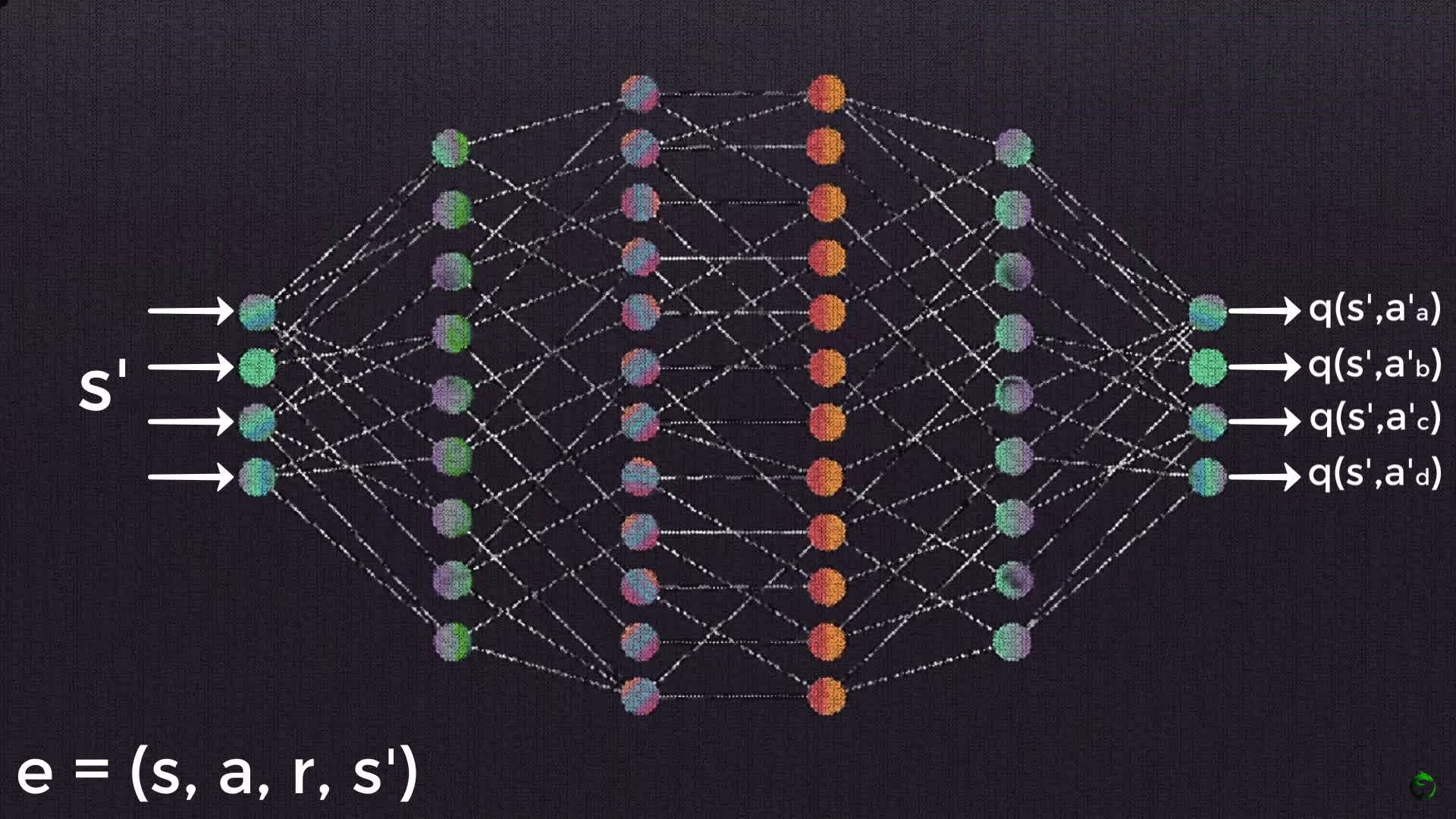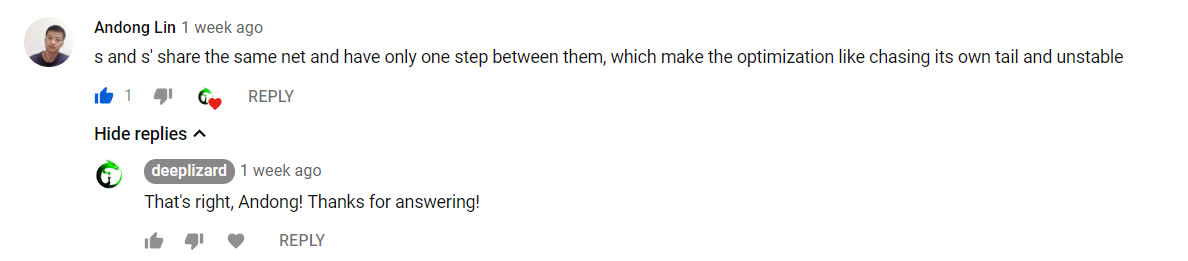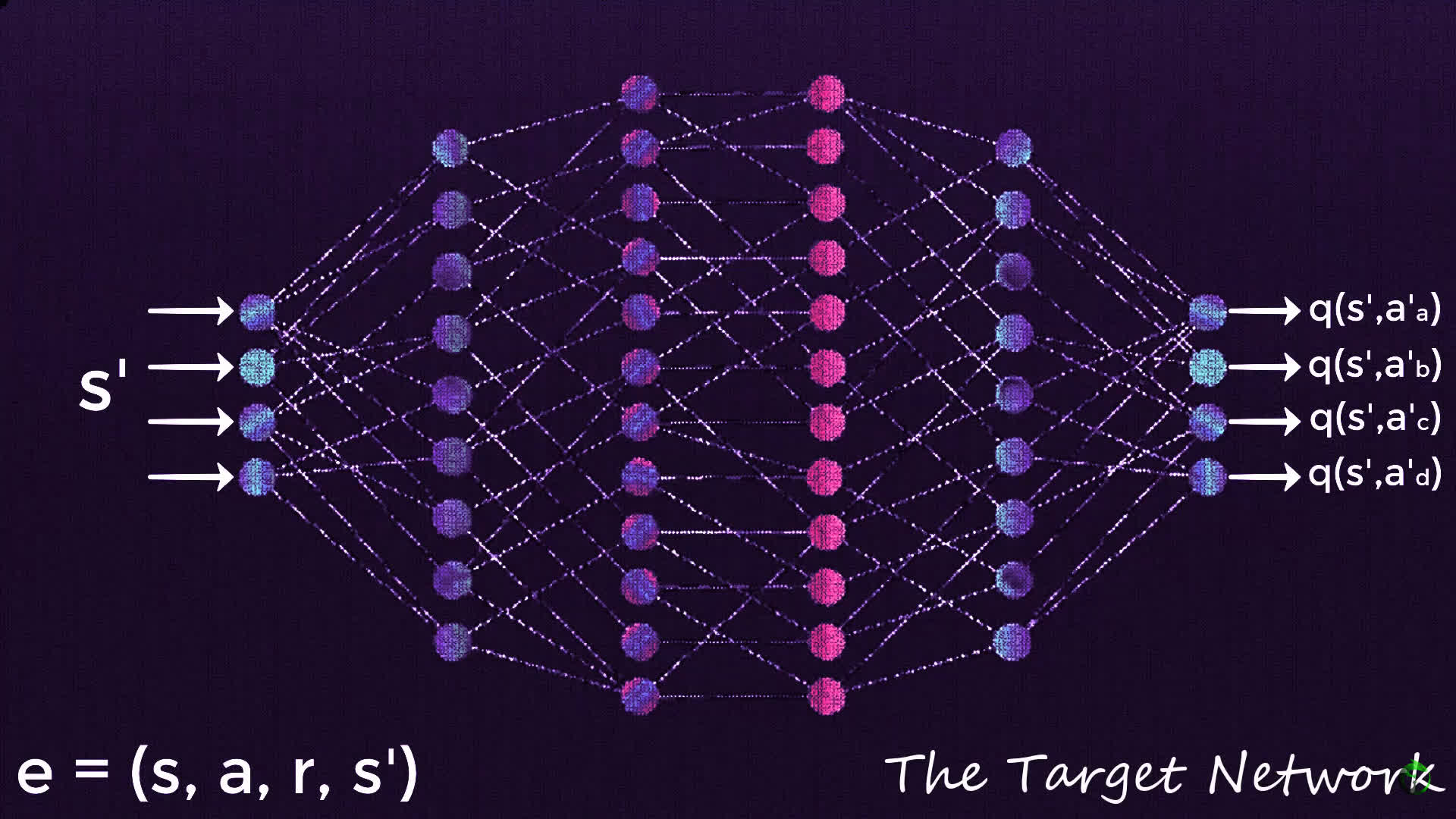# Reinforcement Learning - Goal Oriented Intelligence

Deep Learning Course 4 of 5 - Level: Advanced

## Training a Deep Q-Network with Fixed Q-targets - Reinforcement Learning

### video

expand_more chevron_left

### text

expand_more chevron_left

### Training a deep Q-network with fixed Q-targets

What's up, guys? In this post, we'll continue our discussion of deep Q-networks, and as promised from last time, we'll be introducing a second network called the target network, into the mix. We'll see how exactly this target network fits into the DQN training process. So, let's get to it!### Review: Training the policy network

Recall from last time that we left off with this summary that describes the training process of a deep Q-network.

1. Initialize replay memory capacity.
2. Initialize the network with random weights.
3. For each episode:
1. Initialize the starting state.
2. For each time step:
1. Select an action.
• Via exploration or exploitation
2. Execute selected action in an emulator.
3. Observe reward and next state.
4. Store experience in replay memory.
5. Sample random batch from replay memory.
6. Preprocess states from batch.
7. Pass batch of preprocessed states to policy network.
8. Calculate loss between output Q-values and target Q-values.
• Requires a second pass to the network for the next state
9. Gradient descent updates weights in the policy network to minimize loss.

We briefly mentioned previously that there were some issues that could arise from this approach though. These issues come into play in step $$8$$ where we calculate the loss between the output Q-values and the target Q-values. Remember, this is the step that requires a second pass to the deep Q-network, otherwise known as the policy network.

As a quick refresher, remember, for a single sample, the first pass to the network occurs for the state from the experience tuple that was sampled. The network then outputs the Q-values associated with each possible action that can be taken from that state, and then the loss is calculated between the Q-value for the action from the experience tuple and the target Q-value for this action.To calculate the target Q-value though, we were required to do a second pass to the network with the next state. From this second pass, we can obtain the maximum Q-value among the possible actions that can be taken from that next state, and plug that in to the Bellman equation to calculate the target Q-value for the action from the first pass.

\begin{eqnarray*} q_{\ast }\left( s,a\right)= E\left[ R_{t+1}+\gamma \max_{a^{\prime }}q_{\ast }\left( s^\prime,a^{\prime }\right)\right] \end{eqnarray*}

This process is a bit of a earful, I know, so if you're struggling at all, be sure to spend some time on the previous post where we cover this in full detail.

### Potential training issues with deep Q-networks

Alright, now that we have that refresher out of the way, let's focus on the potential issues with this process. As mentioned, the issues stem from the second pass to the network.

We do the first pass to calculate the Q-value for the relevant action, and then we do a second pass in order to calculate the target Q-value for this same action. Our objective is to get the Q-value to approximate the target Q-value.

Remember, we don't know ahead of time what the target Q-value even is, so we attempt to approximate it with the network. This second pass occurs using the same weights in the network as the first pass.Given this, when our weights update, our outputted Q-values will update, but so will our target Q-values since the targets are calculated using the same weights. So, our Q-values will be updated with each iteration to move closer to the target Q-values, but the target Q-values will also be moving in the same direction.

As Andong put it in the comments of the last video, this makes the optimization appear to be chasing its own tail, which introduces instability. As our Q-values move closer and closer to their targets, the targets continue to move further and further because we're using the same network to calculate both of these values.### The target network

Well, here's a perfect time to introduce the second network that we mentioned earlier. Rather than doing a second pass to the policy network to calculate the target Q-values, we instead obtain the target Q-values from a completely separate network, appropriately called the target network.

The target network is a clone of the policy network. Its weights are frozen with the original policy network's weights, and we update the weights in the target network to the policy network's new weights every certain amount of time steps. This certain amount of time steps can be looked at as yet another hyperparameter that we'll have to test out to see what works best for us.So now, the first pass still occurs with the policy network. The second pass, however, for the following state occurs with the target network. With this target network, we're able to obtain the $$\max$$ Q-value for the next state, and again, plug this value into the Bellman equation in order to calculate the target Q-value for the first state.

$$\max_{a^{\prime }}q_{\ast }\left( s^\prime,a^{\prime }\right)$$
This is all we use the target network for — To find the value of this $$\max$$ term so that we can calculate the target Q-value for any state passed to the policy network.

As it turns out, this removes much of the instability introduced by using only one network to calculate both the Q-values, as well as the target Q-values. We now have something fixed, i.e. fixed Q-targets, that we want our policy network to approximate. So, we no longer have the dog-chasing-it's-tail problem.

As mentioned though, these values don't stay completely fixed the entire time. After $$x$$ amount of time steps, we'll update the weights in the target network with the weights from our policy network, which will in turn, update the target Q-values with respect to what it's learned over those past time steps. This will cause the policy network to start to approximate the updated targets.

### Wrapping up

Alright, so now let's just highlight what's changed in our training summary.

For the most part, this is the same. We only have a few tweaks. The first change is that now we have a new step at the start where we clone the policy network and call it the target network.

Additionally, when we calculate the loss between the Q-values output from the policy network and the target Q-values, we do this using the new target network now, rather than with a second pass to the policy network.

The last change is just that we update the target network weights with the policy network weights every $$x$$ time steps.1. Initialize replay memory capacity.
2. Initialize the policy network with random weights.
3. Clone the policy network, and call it the target network.
4. For each episode:
1. Initialize the starting state.
2. For each time step:
1. Select an action.
• Via exploration or exploitation
2. Execute selected action in an emulator.
3. Observe reward and next state.
4. Store experience in replay memory.
5. Sample random batch from replay memory.
6. Preprocess states from batch.
7. Pass batch of preprocessed states to policy network.
8. Calculate loss between output Q-values and target Q-values.
• Requires a pass to the target network for the next state
9. Gradient descent updates weights in the policy network to minimize loss.
• After $$x$$ time steps, weights in the target network are updated to the weights in the policy network.

Take some time to go over this new algorithm and see if you now have the full picture for how target networks fit into the deep Q-network training process. Let me know your thoughts in the comments! I'll see ya in the next one!

### quiz

expand_more chevron_leftDEEPLIZARD Message notifications

### resources

expand_more chevron_left
Welcome back to this series on reinforcement learning! In this video, we'll continue our discussion of deep Q-networks, and as promised from last time, we'll be introducing a second network called the target network, into the mix. We'll see how exactly this target network fits into the DQN training process, and we'll explore the concept of fixed Q-targets. So, let's get to it! Sources: Reinforcement Learning: An Introduction, Second Edition by Richard S. Sutton and Andrew G. Bartow http://incompleteideas.net/book/RLbook2020.pdf Playing Atari with Deep Reinforcement Learning by Deep Mind Technologies https://www.cs.toronto.edu/~vmnih/docs/dqn.pdf Nick Bostrom TED Talk: https://youtu.be/MnT1xgZgkpk 🕒🦎 VIDEO SECTIONS 🦎🕒 00:00 Welcome to DEEPLIZARD - Go to deeplizard.com for learning resources 00:30 Help deeplizard add video timestamps - See example in the description 07:05 Collective Intelligence and the DEEPLIZARD HIVEMIND 💥🦎 DEEPLIZARD COMMUNITY RESOURCES 🦎💥 👋 Hey, we're Chris and Mandy, the creators of deeplizard! 👀 CHECK OUT OUR VLOG: 🔗 https://youtube.com/deeplizardvlog 👉 Check out the blog post and other resources for this video: 🔗 https://deeplizard.com/learn/video/xVkPh9E9GfE 💻 DOWNLOAD ACCESS TO CODE FILES 🤖 Available for members of the deeplizard hivemind: 🔗 https://deeplizard.com/resources 🧠 Support collective intelligence, join the deeplizard hivemind: 🔗 https://deeplizard.com/hivemind 🤜 Support collective intelligence, create a quiz question for this video: 🔗 https://deeplizard.com/create-quiz-question 🚀 Boost collective intelligence by sharing this video on social media! ❤️🦎 Special thanks to the following polymaths of the deeplizard hivemind: Tammy Prash Zach Wimpee 👀 Follow deeplizard: Our vlog: https://youtube.com/deeplizardvlog Facebook: https://facebook.com/deeplizard Instagram: https://instagram.com/deeplizard Twitter: https://twitter.com/deeplizard Patreon: https://patreon.com/deeplizard YouTube: https://youtube.com/deeplizard 🎓 Deep Learning with deeplizard: Fundamental Concepts - https://deeplizard.com/learn/video/gZmobeGL0Yg Beginner Code - https://deeplizard.com/learn/video/RznKVRTFkBY Intermediate Code - https://deeplizard.com/learn/video/v5cngxo4mIg Advanced Deep RL - https://deeplizard.com/learn/video/nyjbcRQ-uQ8 🎓 Other Courses: Data Science - https://deeplizard.com/learn/video/d11chG7Z-xk Trading - https://deeplizard.com/learn/video/ZpfCK_uHL9Y 🛒 Check out products deeplizard recommends on Amazon: 🔗 https://amazon.com/shop/deeplizard 📕 Get a FREE 30-day Audible trial and 2 FREE audio books using deeplizard's link: 🔗 https://amzn.to/2yoqWRn 🎵 deeplizard uses music by Kevin MacLeod 🔗 https://youtube.com/channel/UCSZXFhRIx6b0dFX3xS8L1yQ 🔗 http://incompetech.com/ ❤️ Please use the knowledge gained from deeplizard content for good, not evil.

expand_more chevron_leftDEEPLIZARD Message notifications